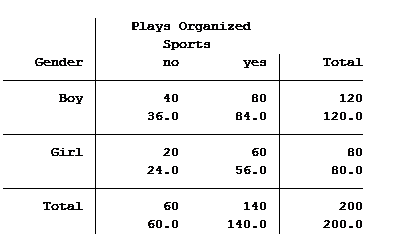# predicted count

### Poisson or Negative Binomial? Using Count Model Diagnostics to Select a Model

March 19th, 2018 by

How do you choose between Poisson and negative binomial models for discrete count outcomes?

One key criterion is the relative value of the variance to the mean after accounting for the effect of the predictors. A previous article discussed the concept of a variance that is larger than the model assumes: overdispersion.

(Underdispersion is also possible, but much less common).

There are two ways to check for overdispersion: (more…)

### Getting Accurate Predicted Counts When There Are No Zeros in the Data

March 12th, 2018 by

We previously examined why a linear regression and negative binomial regression were not viable models for predicting the expected length of stay in the hospital for people with the flu.  A linear regression model was not appropriate because our outcome variable, length of stay, was discrete and not continuous.

A negative binomial model wasn’t the proper choice because the minimum length of stay is not zero. The minimum length of stay is one day. Negative binomial and Poisson models can only be used on data where the observations’ outcome have the possibility of having a zero count.

We need to use a truncated negative binomial model to analyze the expected length of stay of people admitted to the hospital who have the flu. Calculating the expected length of stay is an easy task once we create our model. (more…)

### The Problem with Linear Regression for Count Data

February 26th, 2018 by

Imagine this scenario:

This year’s flu strain is very vigorous. The number of people checking in at hospitals is rapidly increasing. Hospitals are desperate to know if they have enough beds to handle those who need their help.

You have been asked to analyze a previous year’s hospitalization length of stay by people with the flu who had been admitted to the hospital. The predictors in your data set are age group, gender and race of those admitted. You also have an indicator that signifies whether the hospital was privately or publicly run.

### Two-Way Tables and Count Models: Expected and Predicted Counts

December 29th, 2016 by

In a previous article, we discussed how incidence rate ratios calculated in a Poisson regression can be determined from a two-way table of categorical variables.

Statistical software can also calculate the expected (aka predicted) count for each group. Below is the actual and expected count of the number of boys and girls participating and not participating in organized sports.The value in the top of each cell is the actual count (40 boys do not play organized sports) and the bottom value is the expected/predicted count (36 boys are predicted to not play organized sports).

The Poisson model that we ran in the previous article generated the following table: (more…)